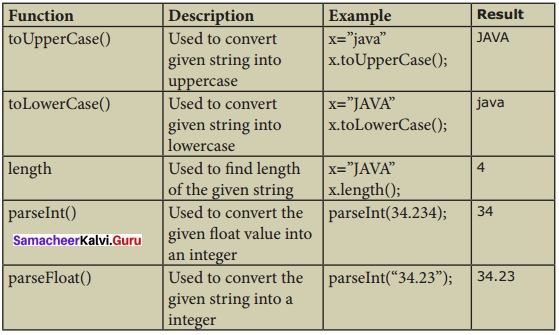Students can Download Computer Applications Chapter Chapter 16 Javascript Functions Questions and Answers, Notes Pdf, Samacheer Kalvi 11th Computer Applications Book Solutions Guide Pdf helps you to revise the complete Tamilnadu State Board New Syllabus and score more marks in your examinations.

## Tamilnadu Samacheer Kalvi 11th Computer Applications Solutions Chapter 16 Javascript Functions

### Samacheer Kalvi 11th Computer Applications Javascript Functions Text Book Back Questions and Answers

Question 1.
The parameters work as:
(a) local variable
(b) global Variable
(c) file variable
(d) block variable
(a) local variableQuestion 2.
Predefined functions are also called as:
(a) library functions
(b) storage functions
(c) instructions
(d) commands
(a) library functions

Question 3.
Larger programs are divided into smaller are called:
(a) modules
(b) block
(c) sets
(d) group
(a) modulesQuestion 4.
Which of the following is used to enhance re-usability and program clarity?
(a) Functions
(b) Modules
(c) Sets
(d) Instructions
(a) Functions

Question 5.
Which of the following allow the programmer to modularize a program?
(a) Library functions
(b) User defined functions
(c) Normal functions
(d) Ordinary functions
(b) User defined functions

II. Answer To The Following Questions

Question 1.
What is a function in JavaScript?
A function is a block of JavaScript code that is defined once but may be executed or invoked any number of times. Functions are used to encapsulate code that performs a specific task.Question 2.
What is the use of function?
Sometimes functions are defined for commonly required tasks to avoid the repetition entailed in typing the same statements over and over. More generally, they are used to keep code that performs a particular job in one place in order to enhance re-usability and program clarity.

Question 3.
Write a note on Library functions?
Pre-defined functions are already defined in the JavaScript library which are also called Library functions. For example isNaN( ), toUpperCase( ), toLowerCase( ), length( ), alert( ), prompt( ),write( ) etc… are pre-defined functions.

Question 4.
Write a note on user defined functions?
User-defined functions allow the programmer to modularize a program. Most computer programs that solve real-world problems are much large, occupy more space in the computer memory and takes more time to execute. Hence such large programs are divided into small programs are called modules.Question 5.
Write the syntax of functions?
The format of a function definition is –
Function function-name(parameters list)
{
Declaration of variable Executable statements;
}

III. Answer To The Following Questions

Question 1.
Write a program in JavaScript to find the cube of a number using function?
<html>
<title> print the cube number
</title>
<Title>
Function cube</Title>
<Script type = “text/Javascript”>
<!—
var input = window.prompt (“Enter value : ”, “0”);
var v = parselnt (input); var c = cube (v);
document, write In (“<br><h4><u> cube function </u> </h4>”);
document, write In (“Number *V* “<br> The cube =” *C);
function cube (x)
{
var cube = x* x * x; return c;
}
//–>
</script>
<body>
</body>
</html>
OUTPUT
Enter value : 5
Cube Function Number : 5
The cube : 125Question 2.
Write a program in JavaScript to find the sum of 10 numbers using function?
<html>
<Title> Function sum of 10 Numbers </Title>
<Script type = “text/Javascript”>
<!—
var input 1 = window.prompt (“Enter initial value : ”, “0”);
var input 2 = window.prompt (“Enter final value : ”, “0”);
var v1 = parse Int (input 1);
var v2 = parse Int (input 2);
var r = res (v1 , v2);
document, write In (“<br><h4><u> Function for sum of 10 Numbers </u> </h4>”);
document, write In (“Initial Number : “ +v1+” <br> Final Number : “+v2+”<br>
The result =”+r);
function result (n)
{
var r = n* (n+1)/2
return r;
//–>
</script>
<body>
</body>
</html>
OUTPUT
Enter initial value : 1
Enterfinal value : 10
Function for sum of 10 numbers
Initial No : 1
FinalNo : 10
The result : 55

### Samacheer Kalvi 11th Computer Applications Javascript Functions Additional Questions and Answers

Question 1.
…………………… often use their argument values to compute a return value that becomes the value of the function invocation expression.
(a) function
(b) parameter
(c) non-parameter
(d) expression
(a) functionQuestion 2.
…………………… method returns the element that has the ID attribute with the specified value.
(a) elements [o]
(b) getElementByid( )
(c) Nan ( )
(d) NaN (“A”)
(b) getElementByid( )

Question 1.
What are the types of function?
JavaScript supports two types functions. They are:

1. Pre-defined or Library Functions
2. User-defined FunctionsQuestion 2.
Define NaN( )?
The is NaN( ) function is used to check whether the given value or variable is valid number. This function returns true if the given value is not a number. For example is NaN(“12”), is NaN(“A”).

Question 3.
What are the different types of predefined functions?III. Explain in Detail

Question 1.
Write a program to find the sum of 2 numbers?
<html>
<title>Function Example</title>
<script type=“text/JavaScript”>
var input1=window.prompt(“Enter Value 1 “0”);
var input2=window.prompt(“Enter Value2 “0”);
var v1=parselnt(input1);
var v2=parselnt(input2);
var s=sum(v1,v2);
document.writeln(“<br><h4><u>Example for Function</u></h4>”);
document.writeln(“First No + v1 + “ <br>Second No + v2 + “<br> The Sum =” + s);
function sum(x, y)
{
var s=x+y;
return s;
}
</script>
<body>
</body>
</html>Question 2.
Write a program to validate whether the entered value is a number or not?
<html>
<title>Example Program to test isNan( ) Function</title>
<body>
<h4><u>Example Program to test isNan() Function</u></h4>
<script language=“JavaScript”>
function checknum( )
{
var n=document.form1.text1 .value;
if(isNaN (n)==true)
{
document.form1.text2.value=“Not a Number +n;
}
else
{
document, form 1 ,text2.value=“It is Number : ”+n;
}
}
</script>
<form name=“form1’’>
Enter a Number 1:
<input type=“text” name=“textl” size=3>
<br><br>
<input type= “button” value=“Click to Check” onClick=“checknum( )”>
<input type=“text” name=“text2” size=30>
<br>
</form>
</body>
</html>Question 3.
Write a program using functions to have an online quiz?
<html>
<title>On-line Quiz</title>
<script type= “text/JavaScript”>
{
//var myQuiz=document.getElementById(“myQuiz”);
if (document.getElementById(“myQuiz”).elements,checked)
else
}
</script>
<body>
<p> Which is not a Programming Language: <br>